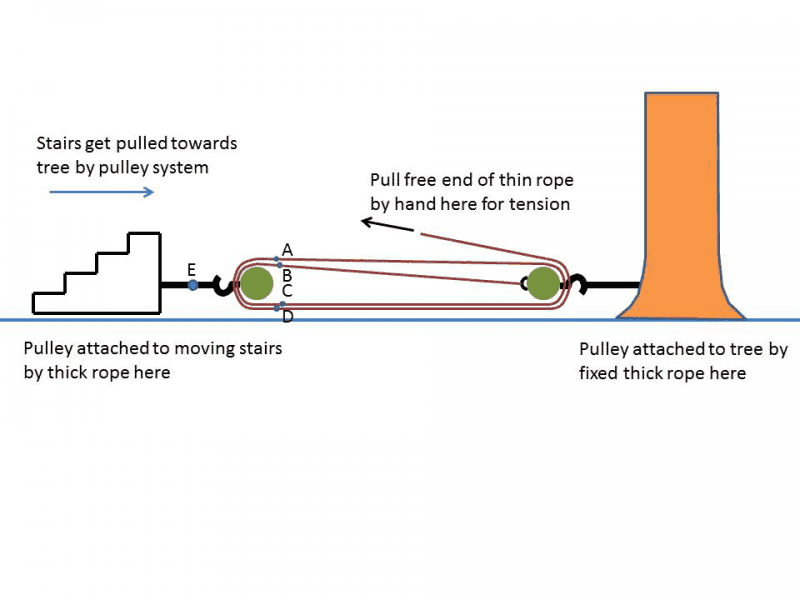# Forces with Tension and pulleys

• Ghost4512

## Homework Statement

1. I had a set of old concrete steps, with a mass of about 2.5 metric tonnes (i.e., 2500 kg), which needed to be dragged across the yard. One way to make the job easier was to rig a block-and-tackle, which consisted of two pulleys. I used a thick rope to tie one pulley to the stairs to be moved. I used another thick rope to tie the other pulley to a large tree. Then, I threaded a thin rope around each pulley twice, as sketched below. Unfortunately, the thin rope was only rated for a maximum tension of 80 lbs., which is 356N. Beyond this point, it began stretching without the tension increasing very much, so let us assume that the maximum rated tension is applied to the thin rope.
a)1. Consider the pulley that is tied to the stairs. If the pulleys are all frictionless, what is the tension in the thin rope at points A, B, C, and D?

2. In view of your answer to part a), draw a free-body diagram for the pulley. (Hint: how many strands of rope are pulling it to the right, and how many ropes are pulling it to the left? You may assume all ropes are approximately horizontal). What must be the tension in the thick rope at point E?

3. Continue to assume that the maximum tension is applied to the thin rope. If the steps are to be dragged at a constant velocity over level ground, what is the maximum allowable coefficient of friction between the steps and the ground?

4. Suppose now that the coefficient of friction is actually 0.15. The maximum amount of tension is still applied to the thin rope. How much additional force, directed horizontally, must be applied towards in order to drag the steps at constant velocity, and is that additional force to the right, or to the left?

Newtons laws

## The Attempt at a Solution

Ok so I don't know if I got these right but my attempt was this so far.

1. So I think A,B,C,D should be the same so I got t=mg/4 =(2500kg*9.80(m/s^2))/4 = 6125 N.
2. My attempt at the FBD pic below The Tension in point E should just be the sum right? So it would be 24500 N.
3. This is where I am stuck Here is where I am at on it. So I don't If I am doing this right or not.

Any help is greatly appreciated.

#### Attachments

1. & 2. Doesn't the problem give you the tension in the thin rope at one end? Wouldn't the answers in 1) and 2) be based on this?

3. Is the block accelerating?

4. Is the additional force being applied on the block directly?

•Ghost4512
Oh, so for 1) the Tension should just be 89N each. And then for 3) the u_k = Tension in E/ mg.

Last edited:
That sounds better.

•Ghost4512
And for the last part I am a little stumped. The additional force would be to the right, but I can not figure out how to actually show that.

Do the same thing as you did in part 3. Make an assumption about the direction and see what answer you get.

•Ghost4512
Thanks for the help! The only thing I screwed up was the first part. It was 356N. For some reason I divided it by 4, but everything else was right.